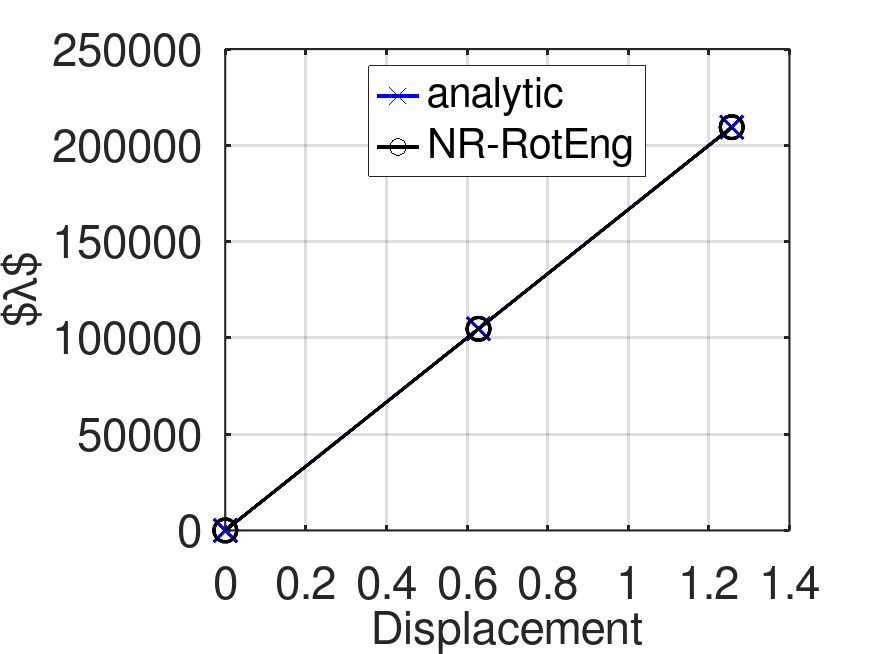# Uniform curvature cantilever beam example

In this tutorial, the Uniform curvature cantilever example and its resolution using ONSAS are described. The aim of this example is to validate the static co-rotational 3D beam implementation by comparing the results provided by ONSAS with the analytical solution. The Octave script of this example is available at this url.

The problem consists in a beam, with one free end (right) submitted to a nodal moment $M$, and the other end (left) constrained (welded), as it is shown in the figure.Before defining the structs, the workspace is cleaned, the ONSAS directory is added to the path and scalar geometry and material parameters are defined.

close all, clear all ;
addpath( genpath( [ pwd '/../../src'] ) );
% material scalar parameters
E = 200e9 ;  nu = 0.3 ;
% geometrical scalar parameters
l = 10 ; ty = .1 ;  tz = .1 ;
% the number of elements of the mesh
numElements = 10 ;

##Analytic solution The rotation of the right end, for a given moment $M$, can be computed as:

$$$M( \theta ) = E I_y \frac{ \theta}{ l } ;$$$

##Numerical solution

### MEBI parameters

The modelling of the structure begins with the definition of the Material-Element-BoundaryConditions-InitialConditions (MEBI) parameters.

### materials

Since the example contains only one rod the fields of the materials struct will have only one entry. Although, it is considered constitutive behavior according to the SaintVenantKirchhoff law:

materials.hyperElasModel  = '1DrotEngStrain' ;
materials.hyperElasParams = [ E nu ] ;

The density is not defined, therefore it is considered as zero (default), then no inertial effects are considered (static analysis).

### elements

Two different types of elements are considered, node and beam. The nodes will be assigned in the first entry (index $1$) and the beam at the index $2$. The elemType field is then:

elements(1).elemType = 'node'  ;
elements(2).elemType = 'frame' ;

for the geometries, the node has not geometry to assign (empty array), and the truss elements will be set as a rectangular-cross section with $t_y$ and $t_z$ cross-section dimensions in $y$ and $z$ directions, then the elemTypeGeometry field is:

elements(2).elemTypeGeometry = [2 ty tz ] ;
elements(2).elemTypeParams   = 1          ;

### boundaryConds

The elements are submitted to two different BC settings. The first BC corresponds to a welded condition (all 6 dofs set to zero)

Iy = ty*tz^3/12 ;
boundaryConds(1).imposDispDofs = [ 1 2 3 4 5 6 ] ;
boundaryConds(1).imposDispVals = [ 0 0 0 0 0 0 ] ;

and the second corresponds to an incremental nodal moment, where the target load produces a circular form of the deformed beam.

boundaryConds(2).loadsCoordSys = 'global'        ;
boundaryConds(2).loadsTimeFact = @(t) E*Iy*2*pi/l *t ;
boundaryConds(2).loadsBaseVals = [ 0 0 0 -1 0 0 ] ;

### initial Conditions

homogeneous initial conditions are considered, then an empty struct is set:

initialConds                = struct() ;

### mesh parameters

The coordinates of the nodes of the mesh are given by the matrix:

mesh.nodesCoords = [ (0:(numElements))'*l/numElements  zeros(numElements+1,2) ] ;

The connectivity is introduced using the conecCell. Each entry of the cell contains a vector with the four indexes of the MEBI parameters, followed by the indexes of the nodes of the element (node connectivity). For didactical purposes each element entry is commented. First the cell is initialized:

mesh.conecCell = { } ;

then the first two nodes are defined, both with material zero (since nodes dont have material), the first element type (the first entry of the cells of the elements struct), and the first entry of the cells of the boundary conditions struct. No non-homogeneous initial condition is considered (then zero is used) and finally the node is included.

mesh.conecCell{ 1, 1 } = [ 0 1 1 0  1   ] ;

the following case only differs in the boundary condition and the node number

mesh.conecCell{ 2, 1 } = [ 0 1 2 0  numElements+1 ] ;

the beam elements are formed by the first material, the second type of element, and no boundary conditions are applied to any element.

for i=1:numElements,
mesh.conecCell{ i+2,1 } = [ 1 2 0 0  i i+1 ] ;
end

### analysisSettings

analysisSettings.methodName    = 'newtonRaphson' ;
analysisSettings.deltaT        =   0.1  ;
analysisSettings.finalTime      =   1    ;
analysisSettings.stopTolDeltau =   1e-6 ;
analysisSettings.stopTolForces =   1e-6 ;
analysisSettings.stopTolIts    =   10   ;

## otherParams

otherParams.problemName = 'uniformCurvatureCantilever';
otherParams.controlDofs = [ numElements+1  4 ] ;
otherParams.plotsFormat = 'vtk' ;

## Analysis case 1: NR with Rotated Eng Strain

In the first case ONSAS is run and the solution at the dof (angle of node B) of interest is stored:

[matUs, loadFactorsMat] = ONSAS( materials, elements, boundaryConds, initialConds, mesh, analysisSettings, otherParams ) ;

the control dof to verificate the solution is the node angle B, this corresponds to the following dof number:

angleControlDof      = (numElements+1)*6 - 2;
controlDispsNREngRot =  -matUs(angleControlDof,:) ;
loadFactorsNREngRot  =  loadFactorsMat(:,2) ;

and the analytical value of the load factors is computed

analyticLoadFactorsNREngRot = @(w) E * Iy * w / l ;

## Verification

verifBoolean = norm( analyticLoadFactorsNREngRot( controlDispsNREngRot) ...
< ( norm( analyticLoadFactorsNREngRot( controlDispsNREngRot) ) * 1e-4 ) ;
lw = 2.0 ; ms = 11 ; plotfontsize = 22 ;
labx = xlabel('Displacement');   laby = ylabel('$\lambda$') ;
print('output/verifCantileverBeam.png','-dpng')verifBoolean = norm( analyticLoadFactorsNREngRot( controlDispsNREngRot) - loadFactorsNREngRot' )  < ( norm( analyticLoadFactorsNREngRot( controlDispsNREngRot) ) * 1e-4 )